Latest Banking jobs   »

# Quantitative Aptitude Quiz For FCI Phase I 2022- 2nd December

Directions (1-5): – In each of these questions, two equations (I) and (II) are given. You have to solve both the equations and give answer
(a) if x>y
(b) if x≥y
(c) if x<y
(d) if x ≤y
(e) if x = y or no relation can be established between x and y

Q1. I.4x²+4x-3=0
II. 4y²-8y+3=0

Q2. I. 11x-13y+48=0
II. 13y+11x=290

Q3. I. 2x+3xy=207
II. 15x=945/y

Q4. I. x²-14x+33=0
II. y²-15y+44=0

Q5. I. 8x²+22x-21=0
II. 18y²+27y-35=0

Direction (6 – 10): In each of the following questions, two equations (I) and (II) are given your have to salve both the equations and give answer.
(a) If x > y
(b) If x ≥ y
(c) If x < y
(d) If x ≤ y
(e) If x = y or no relation can be established between x and y.

Q6. I. x²+9x-22=0
II. 2y² – 7y + 6 = 0

Q7. I. 2y² – 13y – 34 = 0
II. 3x² – 11x – 20 = 0

Q8. I. x^4 = 256
II. y² – 16y + 64 = 0

Q9. I. 9x² – 54x + 77 = 0
II. 12y² – 55y + 63 = 0

Q10. I. (x – 1)² = 121
II. y² – 24y + 144 = 0

Directions (11-15): – In each of the following questions, two equations (I) and (II) are given you have to solve both the equations and give answer.
(a) If x > y
(b) If x ≥ y
(c) If x < y
(d) If x ≤ y
(e) If x = y or no relation can be established between x and y.

Q11. I. 4x² – 8x + 3 = 0
II. 4y² + 4y – 3 = 0

Q12. I. x² – 7x + 10 = 0
II. y² – 10y + 21 = 0

Q13. I. 2x² – 7x + 3 = 0
II. 3y² – y = 0

Q14. I. 20x² + 31x + 12 = 0
II. 3y² + 5y + 2 = 0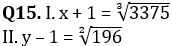Solutions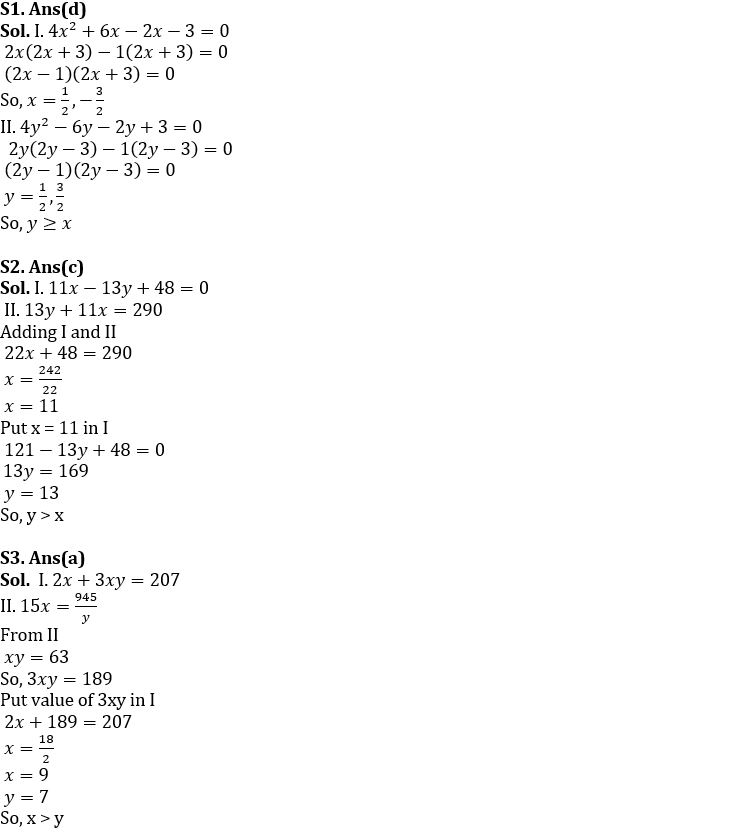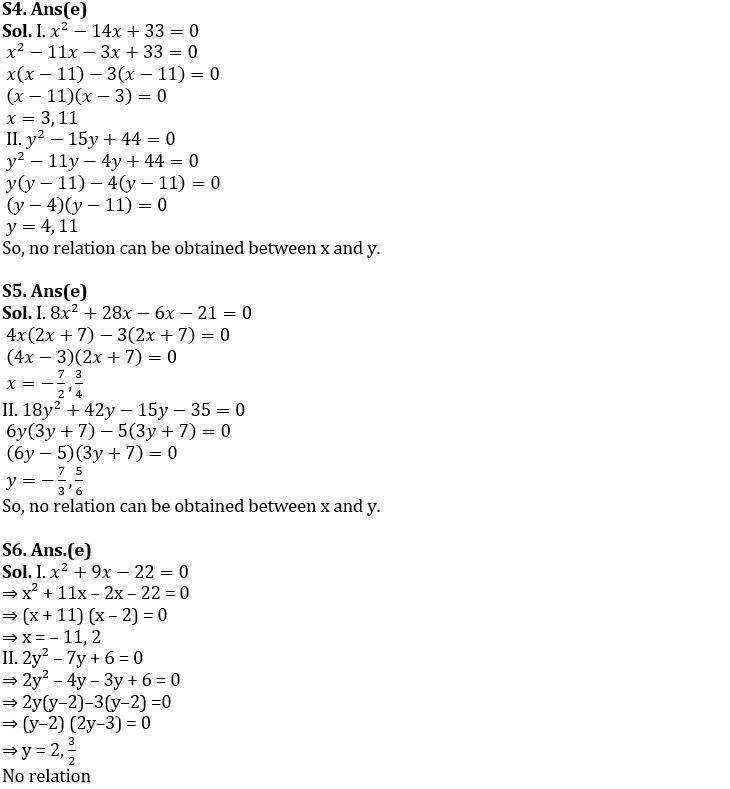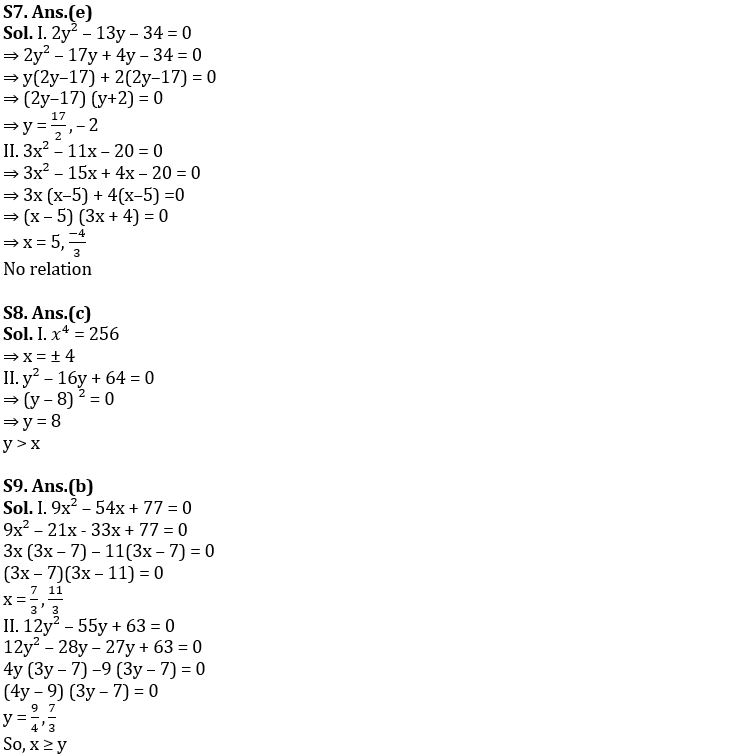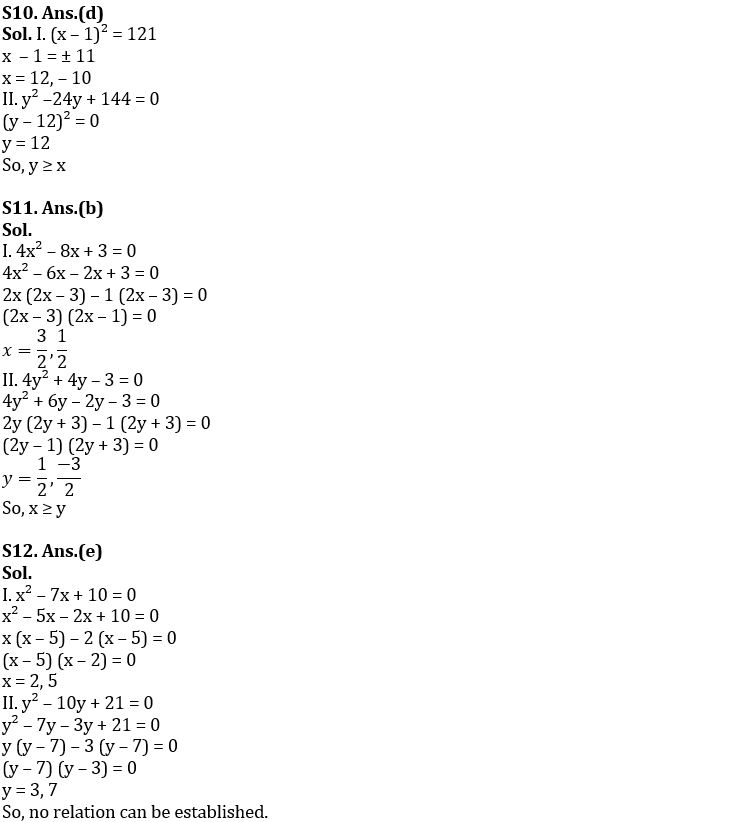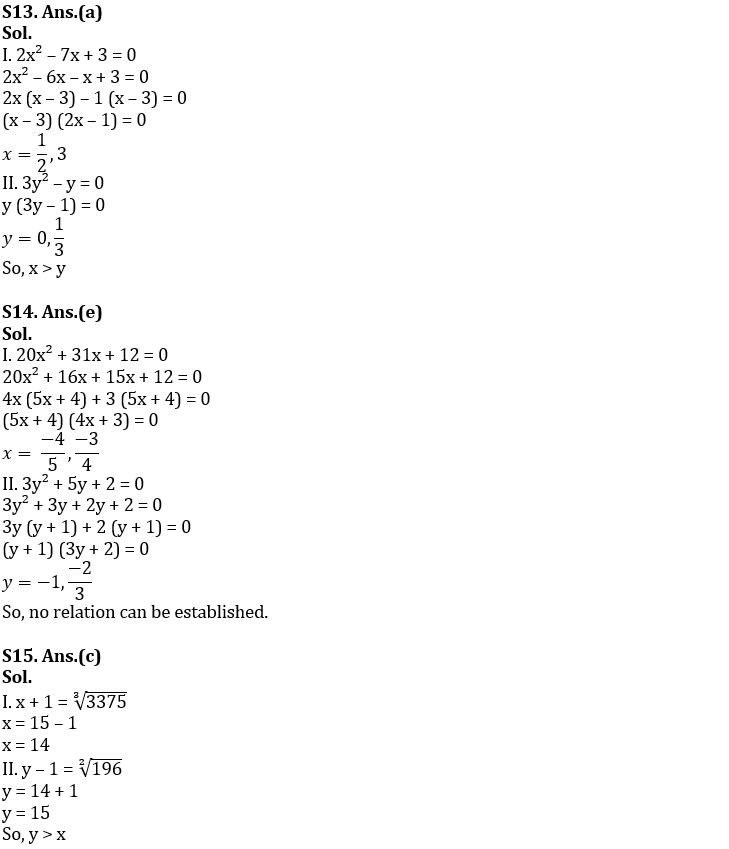#### Congratulations!Union Budget 2023-24: Free PDF